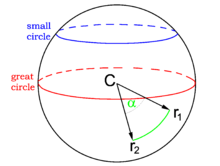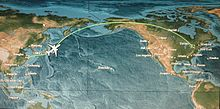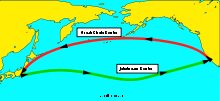# Great circle

﻿
Great circleA great circle divides the sphere in two equal hemispheres

A great circle, also known as a Riemannian circle, of a sphere is the intersection of the sphere and a plane which passes through the center point of the sphere, as opposed to a general circle of a sphere where the plane is not required to pass through the center. (A small circle is the intersection of the sphere and a plane which does not pass through the center.) Any diameter of any great circle coincides with a diameter of the sphere, and therefore all great circles have the same circumference as each other, and have the same center as the sphere. A great circle is the largest circle that can be drawn on any given sphere. Every circle in Euclidean space is a great circle of exactly one sphere.

For any two points on the surface of a sphere there is a great circle through the two points. The minor arc of a great circle between two points is the shortest surface-path between them. In this sense the minor arc is analogous to “straight lines” in spherical geometry. The length of the minor arc of a great circle is taken as the distance between two points on a surface of a sphere, namely the great-circle distance. The great circles are the geodesics of the sphere.

In higher dimensions, the great circles on the n-sphere are the intersection of the n-sphere with two-planes that pass through the origin in the Euclidean space Rn+1.

## Earth geodesics

Strictly speaking the Earth is not a perfect sphere (it is an oblate spheroid or ellipsoid – i.e., slightly compressed at the poles), which means that the shortest distance between two points (a geodesic) is not quite a great circle. The equatorial radius of the earth is about 6378.137 kilometers. The polar radius of the earth is about 6356.752 kilometers (about 21.4 kilometers less). Nevertheless, the sphere model can be considered a first approximation.

When long distance aviation or nautical routes are drawn on a flat map (for instance, the Mercator projection), they often look curved. This is because they lie on great circles. A route that would appear as a straight line on the map would actually be longer. An exception is the gnomonic projection, in which all straight lines represent great circles.

On a spherical Earth, the meridians (or lines of longitude) are great circles, as is the equator. Lines of latitude are not great circles, because they are smaller than the equator; their centers are not at the center of the Earth -- they are small circles instead. Since Earth is not a perfect sphere, the equator (which is generally considered a spherical great circle) is about 40,075 km, while a north-south meridian line (which is an ellipse) is almost 40,008 km. The quadratic mean or root mean square of these extremes provides a decent approximation of the average great-circle circumference, about 40041.5 km.Great-circle route from China to Canada

Some examples of great circles on the celestial sphere include the celestial horizon, the celestial equator, and the ecliptic.

Great circle routes are used by ships and aircraft where currents and winds are not a significant factor. Flight lengths can therefore often be approximated to the great-circle distance between two airports. For aircraft travelling west between continents in the northern hemisphere these paths will extend northward near or into the Arctic region, however easterly flights will often fly a more southerly track to take advantage of the jet stream.

For navigational convenience, Great Circle routes are often broken into a series of shorter rhumb lines which allow the use of constant headings between waypoints along the Great Circle.

## Derivation of shortest pathsAirline routes between San Francisco and Tokyo following the most direct great circle (top), but following the jet stream (bottom) when heading eastwards

To prove that the minor arc of great circle is the shortest path connecting two points on the surface of a sphere, one has to apply calculus of variations to it.

Consider the class of all regular paths from a point p to another point q. Introduce spherical coordinates so that p coincides with the north pole. Any curve on the sphere that does not intersect either pole, except possibly at the endpoints, can be parametrized by$\theta = \theta(t),\quad \phi = \phi(t),\quad a\le t\le b$

provided we allow φ to take on arbitrary real values. The infinitesimal arc length in these coordinates is$ds=r\sqrt{\theta'^2+\phi'^{2}\sin^{2}\theta}\, dt.$

So the length of a curve γ from p to q is a functional of the curve given by$S[\gamma]=r\int_a^b\sqrt{\theta'^2+\phi'^{2}\sin^{2}\theta}\, dt.$

Note that S[γ] does not exceed the length of the meridian from p to q:$S[\gamma] \ge r\int_a^b|\theta'(t)|\,dt \ge r|\theta(q)-\theta(p)|.$

Since the starting point and ending point are fixed, S is minimized if and only if φ' = 0, so the curve must lie on a meridian of the sphere φ = φ0 = constant. In Cartesian coordinates, this is

xsin ϕ0ycos ϕ0 = 0

which is a plane through the origin, i.e., the center of the sphere.

Wikimedia Foundation. 2010.

### Look at other dictionaries:

• great circle — n. any circle described on the surface of a sphere by a plane which passes through the center of the sphere; specif., such a circle on the earth s surface: a course (great circle course) plotted along a great circle of the earth is the shortest… …   English World dictionary

• Great Circle — *Great circle is a circle on the surface of a sphere. *Great Circle is also a fictional organization from Andromeda Nebula, a novel by Ivan Yefremov …   Wikipedia

• great circle — ► NOUN ▪ a circle on the surface of a sphere which lies in a plane passing through the sphere s centre, especially as representing the shortest path between two given points on the sphere …   English terms dictionary

• great circle — noun a circular line on the surface of a sphere formed by intersecting it with a plane passing through the center • Hypernyms: ↑line • Hyponyms: ↑celestial equator, ↑equinoctial circle, ↑equinoctial line, ↑equinoctial, ↑ecliptic, ↑ …   Useful english dictionary

• great circle — A circle on the surface of the sphere (earth) whose center and radii are those of the sphere itself. Only one great circle may be drawn through two places that are not diametrically opposite on the surface of a sphere. The shortest distance… …   Aviation dictionary

• great circle — noun a) A circle defined as the intersection of the surface of a sphere and a plane which passes through the centre of the sphere. Wherever a navigator may be, it certainly would be to his advantage to be able to find the great circle, or… …   Wiktionary

• great circle — great′ cir′cle n. 1) math. a circle on a sphere such that the plane containing the circle passes through the center of the sphere 2) naut. geo a circle of which a segment represents the shortest distance between two points on the surface of the… …   From formal English to slang

• great circle — 1. a circle on a spherical surface such that the plane containing the circle passes through the center of the sphere. Cf. small circle. 2. a circle of which a segment represents the shortest distance between two points on the surface of the earth …   Universalium

• great circle — Synonyms and related words: anklet, aphelion, apogee, armlet, astronomical longitude, autumnal equinox, band, belt, bracelet, celestial equator, celestial longitude, celestial meridian, cincture, cingulum, circle, collar, collarband, colures,… …   Moby Thesaurus

• great circle — noun Date: 1594 a circle formed on the surface of a sphere by the intersection of a plane that passes through the center of the sphere; specifically such a circle on the surface of the earth an arc of which connecting two terrestrial points… …   New Collegiate Dictionary